# What are Oracle Joins (Sql Joins)?

Last updated on March 15th, 2019 at 03:53 pm

Sometimes you need to display data from multiple tables. In order to perform that you need to link one table to another table.There are various ways in which we can obtain data from Multiple tables.Here I would be focusing on Oracle Joins with examples.This is valid for in general any Sql Joins also. I would be explaining inner join,outer join,cross join ,natural join,Cartesian join with examples

## Oracle Joins

A Oracle Joins ( Sql Joins) is a query that combines rows from two or more oracle tables.

Oracle performs a join whenever multiple tables appear in the FROM clause. In order to join two tables, you need to identify the common columns that relate the two tables. In the WHERE clause you define the relationship between the tables listed in the FROM clause.

Some Guidelines for  Oracle Joins ( Sql Joins)

1) when writing the select statement that joins tables, it is good practice to precede the column name with table name for clarity purpose.

2) To join m tables, we need at least m-1 conditions

3) We can use table alias for the tables name if the table name is lengthy. Table looks the code shorter and hence less memory

4)  If you are not using table_name or tables alias while selecting the column and If multiple tables are having same column name, then we should specify the table_name for the column which is common across the tables

## Inner Join

The most common operator used to relate two tables is the equality operator (=). This is called an equality join or equijoin. This simple join also known as inner join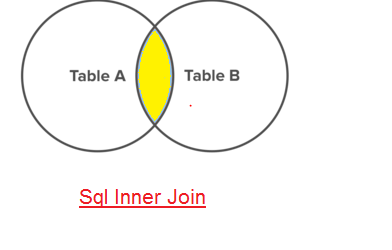#### Oracle Inner Join Example

Syntax
Select tab1.col,tab2.col
from tab1 ,tab2
where tab1.col=tab2.col

SELECT EMP.EMPNO,EMP.ENAME, DEPT.DEPTNO,DEPT.DNAME
FROM EMP , DEPT
where EMP. DEPTNO= DEPT. DEPTNO;

To execute a join of three or more table, Oracle joins two tables based on the join condition, then joins the result to another table, based on join conditions and repeats until all tables are joined.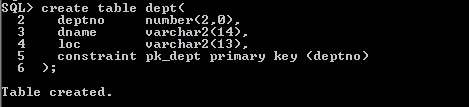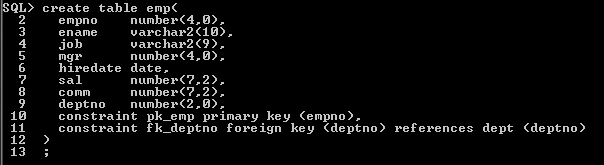## Complex Joins

Have one or more conditions added in the WHERE clause. For example, if you are interested in all department and their locations outside the UK use

SELECT LOCATIONS.LOCATION_ID, CITY, DEPARTMENT_NAME
FROM LOCATIONS, DEPARTMENTS
WHERE LOCATIONS.LOCATION_ID = DEPARTMENTS.LOCATION_ID
AND COUNTRY_ID != ‘UK’;

## Non –equijoin

A non-equijoin is the join condition other than equality operator.

It is an inner join statement that uses an unequal operation (i.e.: <>, >, <, =, BETWEEN, etc.) to match rows from different tables

SELECT e.ename, e.sal, s.grade
FROM  emp e, sal_grade s
WHERE e.sal BETWEEN s.low_sal AND s.high_sal;

## Cartesian Products or Cartesian Join or Cross Join

-A Cartesian join (or Cartesian product)  (or Cross Join) occurs when data is selected from two or more tables and join condition is defined or no common relationship is defined in the WHERE clause

-If a join is performed and no join condition is specified, a Cartesian product results. A Cartesian product is a result set that is the product of the two tables’ total rows. If table “P” has 100 rows and table “Q” has 100 rows and a Cartesian product is developed, the resulting result set will have 10000 rows. If a query joins three or more tables, the optimizer may find a way to choose a join order that precludes a Cartesian product, but don’t count on it.

SELECT EMPNO,ENAME, DEPT.DEPTNO,DNAME  FROM EMP   , DEPT ;
or
SELECT EMPNO,ENAME, DEPT.DEPTNO,DNAME  FROM EMP   cross join DEPT ;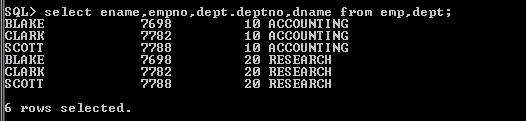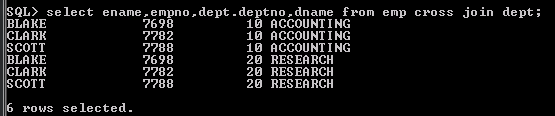## Outer Joins

The inner joins we have seen, return the matching rows from two or more tables according the join condition. The Outer Join mechanism returns the data from one table even if there is no corresponding row in the joining table.

There are three outer joins

a) Left outer Join or Left Join

b) Right outer Join or Right join

c) Full outer join or full join

### Left Outer Join

returns the rows matching from both tables as well as the unmatched rows from the table on the left of the join clause.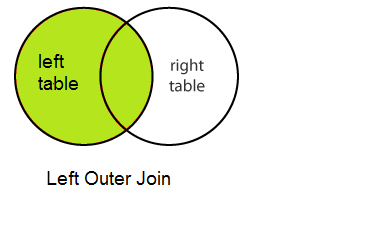The LEFT OUTER JOIN would return the all records from left table and only those records from right table that intersect with right table

Here is the example of Oracle Left outer Join

Example:
select empno,ename,emp.deptno,dname
from emp
LEFT OUTER JOIN dept
on emp.deptno=dept.deptno;

oracle join syntax (+) :In  Oracle database ,we can also used  the plus sign syntax for left outer join.In this case plus join is on the right side of the equation.

Example:
select empno,ename,emp.deptno,dname
from emp ,dept where emp.deptno=dept.deptno(+) ;

### Right Outer Join

returns the rows matching from both tables as well as the unmatched rows from the table on the right of the join clause.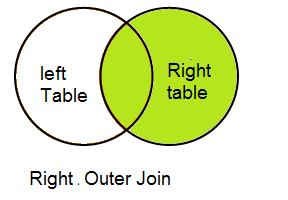Here is the example for Oracle Right outer join

Example:
select empno,ename,dept.deptno,dname
from emp
right OUTER JOIN dept
on emp.deptno=dept.deptno;

oracle join syntax (+) :In  Oracle database ,we can also used  the plus sign syntax for RIGHT OUTER JOIN.In this case right join is indicated when the plus sign is on the left of the equation.

Example:
select empno,ename,dept.deptno,dname
from emp ,dept where emp.deptno(+)=dept.deptno ;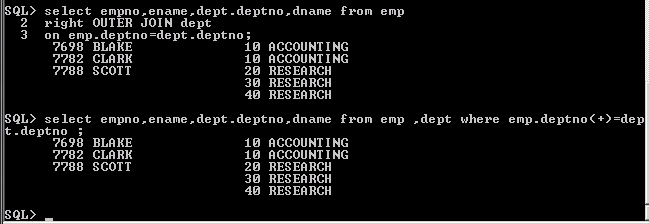### Full Outer Join

This type of join returns all rows from the LEFT-hand table and RIGHT-hand table with nulls in place where the join condition is not met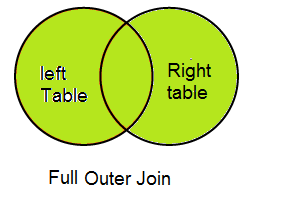Here is the example for Oracle Full Outer Join

select empno,ename,dept.deptno,dname
from emp
full OUTER JOIN dept
on emp.deptno=dept.deptno;

This query will return all rows from both tables. Using Oracle syntax using E.DEPT_ID (+) = D.DEPT_ID (+) is impossible because the (+) sign may reference only one table.  We have to use Union to achieve the same using + sign

select empno,ename,dept.deptno,dname
from emp ,dept where emp.deptno=dept.deptno(+)
union
select empno,ename,dept.deptno,dname
from emp ,dept where emp.deptno(+)=dept.deptno;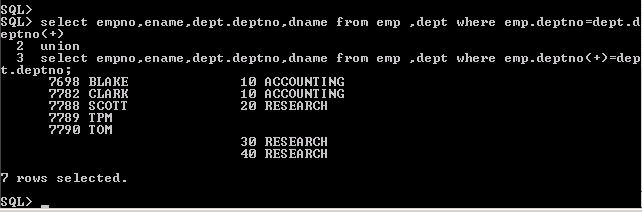Some RDBMS does not support the Full outer join clause ,then we can use below to cover that

select empno,ename,dept.deptno,dname
from emp
left OUTER JOIN dept
on emp.deptno=dept.deptno
union
select empno,ename,dept.deptno,dname
from emp
right OUTER JOIN dept
on emp.deptno=dept.deptno;

The natural join specifies that the join relate all columns with the same names on the two tables.

Basically it compares the common columns of both tables with each other. One should check whether common columns exist in both tables before doing a natural join.

Example

SELECT EMP_ID,EMP_NAME, DEPT_ID,DEPARTMENT_NAME  FROM EMP  NATURAL JOIN DEPT;

Here dept_id is the same column between the tables emp and dept

Natural join can join more than two tables

Hope you like this post on Oracle joins with examples.

Related Articles
sql tutorial

Sql Subqueries : Single, Multiple, Scalar and Correlated

Sql Set Operators: Union,Union All,Intersect and Minus

Complete Guide on Oracle views (Sql Views)

how to write sql queries

Auto Increment Column – Sequence as Default Value in Oracle and mysql

Cross Join in Oracle

self join in oracle with examples

oracle join syntax examples